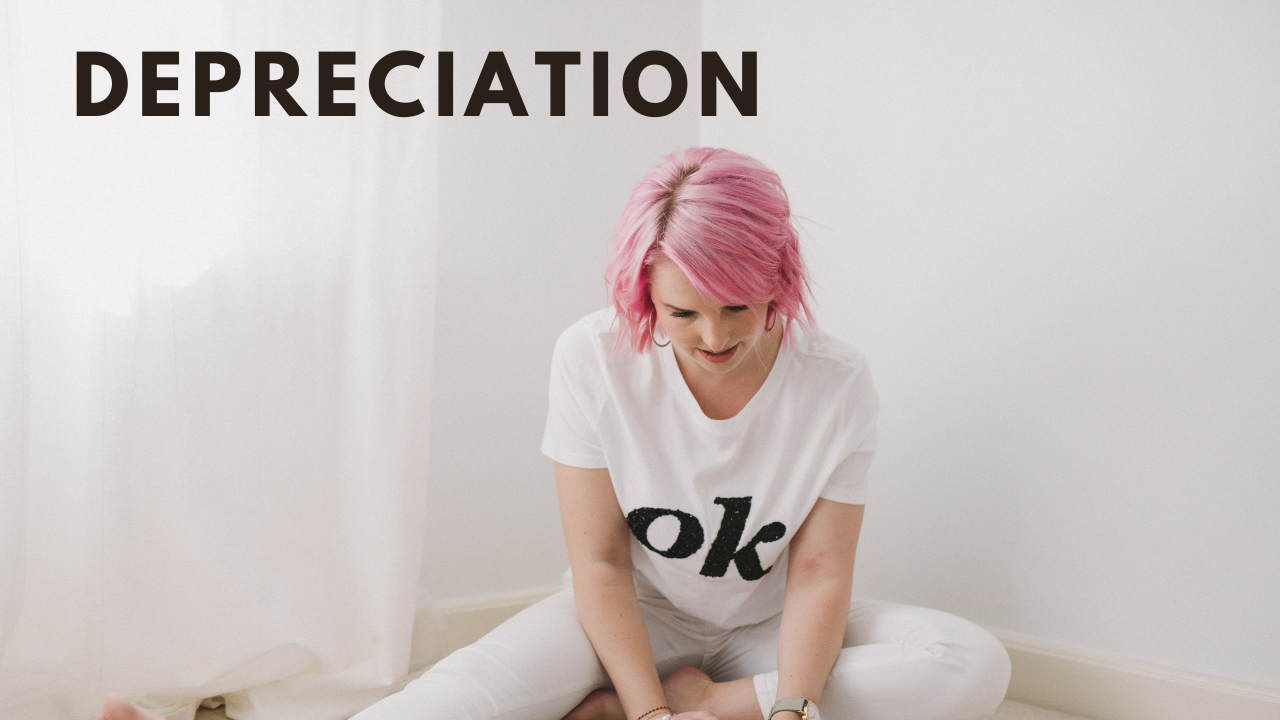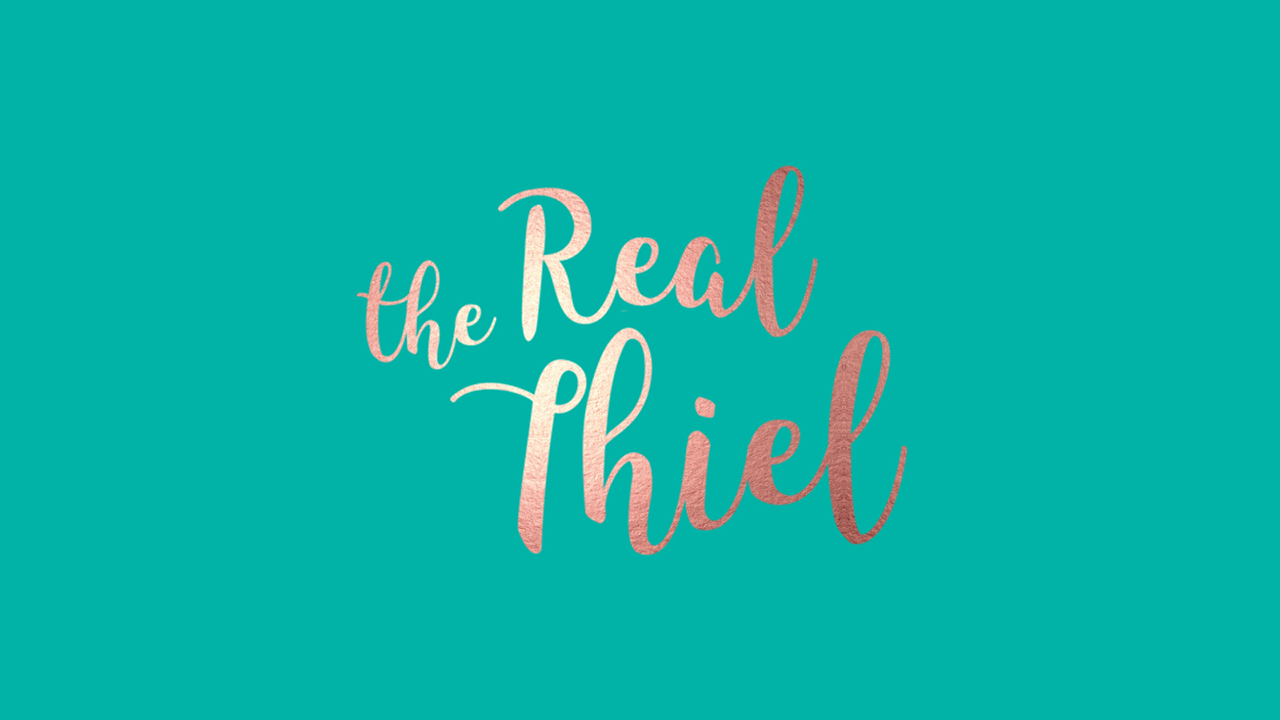Get the e-book: 'Money talks - but what on earth is it saying?'

# Depreciation

Jul 12, 2020I appreciate you… and I depreciate your assets.

## What is Depreciation?

Depreciation is how we account for an assets value becoming reduced through time and use. It is the measure of decline in value of an asset used by a business to generate income. Assets depreciate at different rates and different timelines, and a business can claim depreciation expense as a tax deduction, which reduces the amount of tax you need to pay.

## What can I depreciate?

A depreciable asset is an asset that has a limited effective/useful life and will decline in value over time, commonly assets like office equipment, vehicles, computers & mobile phones - assets purchased to help a business generate income.

If you are not sure whether to depreciate your newly acquired asset, or to deduct for the whole lot in one go, then speak to your friendly accountant.

Key Calculation

Purchase Cost
(-) Accumulated Depreciation
(-) Depreciation Expense for this year
= Asset Value (closing) this is also known as ‘written down value’

You get a deduction in the tax return for the depreciation expense amount, so we need to know how to calculate this depreciation.

## Common Terms

What is the cost of a depreciable asset?

The total cost of a depreciable asset includes its purchase price (excluding GST) and any costs incurred directly in purchasing the asset (i.e. insurance, shipping and handling costs, freight) and getting it ready for use.

What is Accumulated Depreciation?

Accumulated depreciation is the total amount of depreciation claimed since the asset was purchased. For example, if you have claimed \$5,000 in depreciation since you bought your camera 3 years ago, then your accumulated depreciation is \$5,000.

What is Written Down Value/Residual Value?

Written Down value is the value of the asset once you take into account its accumulated depreciation. If you purchased a laptop for \$5,000 and you have accumulated depreciation of \$2,000, the written down value of the laptop is \$3,000 (\$5,000 - \$2,000).

What is Effective Life?

The effective life of an asset is how long it can be used to produce income. Every asset’s effective life varies. For example, the effective life of a laptop is much less than the effective life of a car.  The effective life of an asset is used to calculate depreciation. The ATO has guides for us to use when figuring out the effective life of assets for tax purposes.

So, the big question is, how do we calculate the depreciation expense?

There are 2 methods - ‘Diminishing Value’ and ‘Straight Line’ depreciation

## Calculation Methods

What is Diminishing Value?

Under this method, it is assumed that most of the value of the asset is used up in the first years of its effective life, therefore you receive a greater depreciation deduction in the first years and the deduction will decrease as the years go on.

Diminishing Value depreciation method example

Assuming the asset is 100% for business purposes…

Your business purchased a car for \$40,000 on 1 July 2018. You decide to use the diminishing value depreciation method, and the depreciation rate is 20%. Below is a table demonstrating how depreciation is calculated for the first three years of the asset’s effective life:

 Year Opening Cost Depreciation Rate Depreciation Amount Closing Cost 1 \$40,000.00 20% \$8,000.00 \$32,000.00 2 \$32,000.00 20% \$6,400.00 \$25,600.00 3 \$25,600.00 20% \$5,120.00 \$20,480.00

As you can see, the biggest depreciation amount is gained in the first year of the asset’s life, and the amount ‘diminishes’ (hence diminishing value) over the following years.

What is Straight Line Depreciation?

Straight Line depreciation sees the same depreciation deduction claimed every year.  If an asset was purchased for \$5,000, with an effective life of 5 years, the business will claim \$1,000 (\$5,000 5 years) as a depreciation deduction for five years.

Straight Line depreciation method example

Your business purchases a desk for \$40,000 on 1 July 2018. You decide to use the straight line depreciation method, and the depreciation method is 20%. Below is a table demonstrating how depreciation is calculated for the first three years of the asset’s effective life:

 Year Opening Cost Depreciation Rate Depreciation Amount Closing Cost 1 \$40,000.00 20% \$8,000.00 \$32,000.00 2 \$32,000.00 20% \$8,000.00 \$24,000.00 3 \$24,000.00 20% \$8,000.00 \$16,000.00

As you can see, the same amount is depreciated every year.

## Small Business Instant Write Off

At the time of writing, the ATO permits small businesses, with an aggregated turnover less than \$500 million to instantly write-off asset purchases costing less than \$150,000. However, there are many conditions around the instant write-off, so we wrote a whole blog on it!

## How does my accounting software calculate depreciation?

If you are a business who uses accounting software (if not, we need to talk!), then it will take care of the depreciation calculations for you. Now of course, it needs some TLC and clicks from you (or us if you pay us to do your bookkeeping!), so we wrote a whole blog on what’s called the Fixed Asset Register

So, while your value only increases over time, some of your assets may not. Best to recognise that as an expense and get the deductions you are entitled to!

Xxx Lauren

(and co-author Caitie Copley)

Close50% Complete